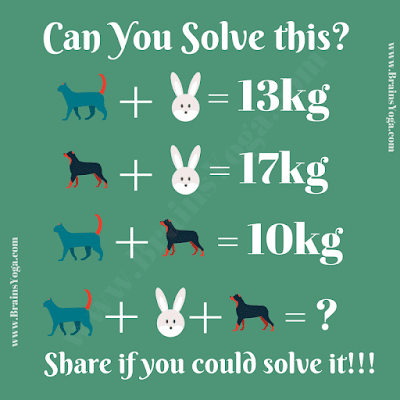Here is Mathematical Brain Teaser in which your challenge is to solve the simple equations. Can you solve these simple Maths equations and find the value of both triangle and star?Can you solve this Maths Equation?